## November 29, 2012

So far I've posted 9 articles here covering different techniques for making OpenGL shaders.

For those of you that have been following and trying things out, I thought this might be a good place for a recap.

I introduced the different built-in functions of GLSL as we needed them to achieve different goals, but at some point you might have been wishing for a compact reference to all the available functions.

The one I use very often is the Khronos Group's 4 page PDF format OpenGL ES 2.0 Quick Reference Card. Page 3 and 4 cover GLSL including all the built-in functions.

There is also an online OpenGL ES Shading Language reference page, which covers also the latest version 3.0 (most devices and WebGL still use the 1.0 version).

Now you have those under your belt, here is a list of all the functions we've used so far.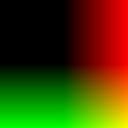```f = vec4(c.x, c.y, 0.0, 1.0);
```

Function: vec2(float,float), vec3(float,float,float), vec4(float,float,float,float)

Description:

Vectors are groups of numbers. These constructor functions are used to create them - pass single numbers or vectors as parameters. For example, vec4(colour.rgb,1.) creates a new vec4 using the first 3 elements of the vector colour and the number 1.0 as the fourth element.Single or multiple elements can be referred to using dot notation using rgba, xyzw or stuv. For example v.xy would return a vec2 containing the x and y elements of vector v (which could be a vec2, vec3 or vec4).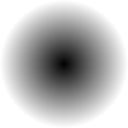```float d = length(c.xy);
f = vec4(d, d, d, 1.0);
```

Function: length(vec)

Description:

Returns the length of the given vector. The length of 2 dimensional vec2 v would be equivalent to sqrt(v.x*v.x+v.y*v.y). Can be used to create a distance field for drawing circles and related shapes.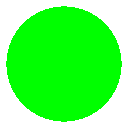```float d = step(0.1, 1.0-length(c.xy));
f = vec4(0.0, 1.0, 0.0, d);
```

Function: step(threshold,value)

Description:

Returns 0.0 if value is less than threshold, otherwise returns 1.0. Used to break a continuous field into two distinct regions.

For example, it can be applied to a distance field calculated using length to create a circle.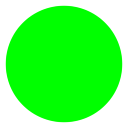```float d = smoothstep(0.08,0.1,1.-length(c.xy));
f = vec4(0.0, 1.0, 0.0, d)
```

Function: smoothstep(lower,upper,value)

Description:

Returns 0.0 if value is less than lower, 1.0 if value is above upper, and smoothly interpolates the range between.

Interpolation is not straight/linear. It uses a function like f = f * f * (3.0 - 2.0 * f)

Used to break a continuous field into two distinct regions with a smooth transition.

For example, it can be applied to a distance field calculated using length to create a circle with smooth or blurred edges.

The greater the gap between lower and upper, the wider the transition region.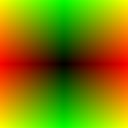```vec2 r=abs(c.xy);
f=vec4(r,0.,1.)
```

Function: abs(value)

Description: Returns the value unchanged if value is greater than zero, or -value if value is less than zero.

The effect is to make continuous values/fields reflect around zero.

Can be used in combination with mod or fract to help make continuous repeating functions.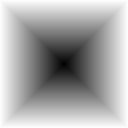```vec2 r=abs(c.xy);float s=max(r.x,r.y);
f=vec4(vec3(s),1.)
```

Function: max(first,second)

Description: Returns first if first is larger than second, else returns second.

Useful for creating squares (or cubes in 3D).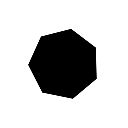```int N=7;
float a=atan(c.x,c.y)+.2;
float b=6.28319/float(N);
f=vec4(vec3( smoothstep(.5,.51, cos(floor(.5+a/b)*b-a)*length(c.xy))),1.);
```

Function: atan(x,y)

Description: Returns the angle, in radians, between +pi and -pi, formed by the right-angled triangle with width x and height y.

Combined with length, it converts cartesian coordinates to polar coordinates.

Can be used for partioning space into regions based on their angle around a centre point.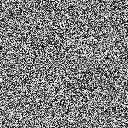```vec2 r=(456.789* sin(789.123*c.xy));
f=vec4(fract( r.x*r.y*(1.+c.x)))
```

Function: sin(value),cos(value),tan(value)

Description: sin and cos are continuous smooth functions in the range -1 to +1 which repeat over a period of 2*Pi. tan (equal to sin divided by cos) also repeats but is not continuous - or limited in range.

These functions are very useful for creating smooth variation, or with high frequencies, pseudo-random aliasing noise.

Depending on your platform, they can be quite expensive to calculate.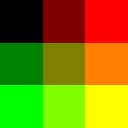```vec2 s=floor(3.*(c.xy*.5+.5))/2.;
f=vec4(s.x,s.y,0.,1.)
```

Function: floor(value)

Description: Returns the integer part of value, discarding the fractional part.

Used for partitioning continuous functions into regions sharing a common constant value which can be used to give all pixels in that region similar behaviour.

Combined with fract to split a value into two parts, it can be used for interpolating between regions.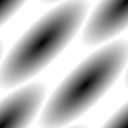```vec2 m=mod(c.xy+c.x+c.y,2.)-1.;f=vec4(length(m))
```

Function: mod(value, period)

Description: Returns the remainder of value divided by period.

Mod can be used to create a repeating function from a continuous function.

fract can be a cheaper alternative.```vec3 sky=mix( vec3(.0,.0,.5), vec3(.0,.5,1.), c.y*.5+.5);
float circle=smoothstep(.25, .3,1.-length(c.xy));
```

Function: mix(first,second,value)

Description: Returns first if value is 0 or less. Returns second if value is 1 or more. Between 0 and 1, returns a linear interpolated blend of first and second.

Mix is used for combining multiple overlapping regions. It can be used to select colours or shapes. Chaining mix results can create complex scenes without needing any conditional statements.```float s=1.-length(c.xy*c.xy*c.xy*c.xy);
f=vec4(1.,.8,.6,smoothstep(0.,0.1,s));
```

Function: pow(value,raise)

Description: Returns value raised to the power raise. Raise can be integers - 2.0 would return value*value - or floats - 0.5 would return sqrt(value).

Useful for making squircles.

Can be an expensive function.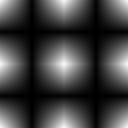```vec2 d=abs(fract(c.xy)*2.-1.);
f=vec4(d.x*d.y)
```

Function: fract(value)

Description: Returns the fractional part of value. Paired with floor, it breaks numbers into two parts - integer and fractional.

Paired with abs, it can create a repeating triangle wave which oscillates linearly between 0 and 1 - sometimes a cheaper alternative to sin.

Very useful for creating aliasing noise.

See More noise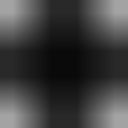```vec2 d = clamp(abs(c.xy),0.2,0.8);
f = vec4(d.x*d.y);
```

Function: clamp(value,min,max)

Description: If value is less than min, returns min. If value is more than max, returns max. Otherwise returns value. Effects is to limit value to the range min-max.

Sometimes the output of one function can exceed the useful input range of another. clamp can constrain the range in this case.

One example is when doing calculations with colours. Display will naturally clamp to the range 0-1, but intermediate calculations using higher or lower values can lead to incorrect results which explicit clamping can correct.